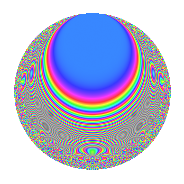# Properties

 Label 108.5.fLevel 108 Weight 5 Character orbit f Rep. character $$\chi_{108}(19,\cdot)$$ Character field $$\Q(\zeta_{6})$$ Dimension 44 Newform subspaces 1 Sturm bound 90 Trace bound 0

# Related objects

## Defining parameters

 Level: $$N$$ $$=$$ $$108 = 2^{2} \cdot 3^{3}$$ Weight: $$k$$ $$=$$ $$5$$ Character orbit: $$[\chi]$$ $$=$$ 108.f (of order $$6$$ and degree $$2$$) Character conductor: $$\operatorname{cond}(\chi)$$ $$=$$ $$36$$ Character field: $$\Q(\zeta_{6})$$ Newform subspaces: $$1$$ Sturm bound: $$90$$ Trace bound: $$0$$

## Dimensions

The following table gives the dimensions of various subspaces of $$M_{5}(108, [\chi])$$.

Total New Old
Modular forms 156 52 104
Cusp forms 132 44 88
Eisenstein series 24 8 16

## Trace form

 $$44q + q^{2} - q^{4} + 2q^{5} - 122q^{8} + O(q^{10})$$ $$44q + q^{2} - q^{4} + 2q^{5} - 122q^{8} + 28q^{10} - 2q^{13} - 252q^{14} - q^{16} + 56q^{17} + 140q^{20} - 33q^{22} - 1752q^{25} - 1096q^{26} - 516q^{28} - 526q^{29} + 121q^{32} + 385q^{34} - 8q^{37} - 1395q^{38} - 2276q^{40} + 2762q^{41} - 6714q^{44} + 3576q^{46} + 3428q^{49} - 6375q^{50} + 1438q^{52} + 10088q^{53} + 7506q^{56} - 4064q^{58} - 2q^{61} + 18324q^{62} + 9026q^{64} + 2014q^{65} + 11405q^{68} + 3666q^{70} - 3416q^{73} - 14620q^{74} + 1581q^{76} + 3942q^{77} - 45520q^{80} - 8486q^{82} - 1252q^{85} - 22113q^{86} + 1995q^{88} - 13048q^{89} + 30294q^{92} + 7524q^{94} + 5638q^{97} + 92938q^{98} + O(q^{100})$$

## Decomposition of $$S_{5}^{\mathrm{new}}(108, [\chi])$$ into newform subspaces

Label Dim. $$A$$ Field CM Traces $q$-expansion
$$a_2$$ $$a_3$$ $$a_5$$ $$a_7$$
108.5.f.a $$44$$ $$11.164$$ None $$1$$ $$0$$ $$2$$ $$0$$

## Decomposition of $$S_{5}^{\mathrm{old}}(108, [\chi])$$ into lower level spaces

$$S_{5}^{\mathrm{old}}(108, [\chi]) \cong$$ $$S_{5}^{\mathrm{new}}(36, [\chi])$$$$^{\oplus 2}$$

## Hecke characteristic polynomials

There are no characteristic polynomials of Hecke operators in the database